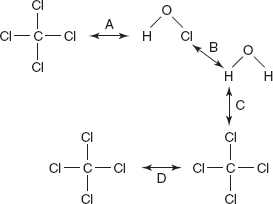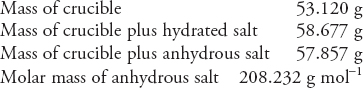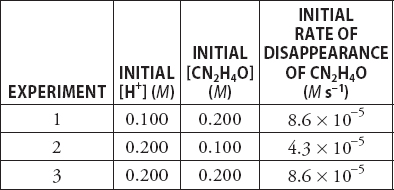# AP Chemistry Practice Test 42

### Test Information12 questions18 minutes

1.Which of the labeled arrows in the diagram above represents the strongest intermolecular force?

`Questions 2-5 refer to the following information.A student builds a Galvanic cell to take advantage of the following reaction:Zn(s) + 2 AgNO3(1 M) → 2 Ag(s) + Zn(NO3)2(1 M)The cell's voltage is measured and found to be +1.56 volts.`

2. What happens to the voltage when a small amount of silver nitrate is added to the silver compartment?

3. What happens to the cell voltage when the student replaces the Zn(NO3)2 solution with 1 M ZnCl2?

4. If the standard reduction potential of silver is 0.80 V, what is the standard reduction potential of zinc?

5. What happens to the cell voltage after the cell has reached equilibrium?

`Questions 6-10 refer to the following information.Many metal salts crystallize from solution in the form of a hydrate. If the formula of the anhydrous salt is AaXx, then the generic formula of the hydrated form would be AaXx•xH2O. A student conducts an experiment to determine the formula of a metal oxide by collecting the following data:The hydrated salt is finely powdered to ensure complete water loss to produce the anhydrous salt.`

6. How many grams of water were in the hydrate?

7. What is the approximate percent water in the hydrated salt?

8. The student did not have time to finish the experiment during the lab period and was forced to store the anhydrous salt and crucible in his lab drawer until the next day. At the beginning of the next lab period the student weighed the anhydrous salt and beaker. After calculating the percent water in the sample, the student found that the percentage was lower than predicted. Assuming all weighing were done correctly, what might be the cause of the lower than expected percentage?

9. In another experiment on a different metal hydrate a student found that the salt was 62.9% water. In this case, the molar mass of the anhydrous salt was 106 g mol-1. Which of the following general formulas gives the correct value of x?

10. Manganese normally forms one of four oxides. The oxides are MnO, Mn2O3, MnO2, and Mn3O4. Which of the four oxides has the highest percentage of oxygen? (Molar masses: O = 16.0 g mol-1, Mn = 54.9 g mol-1, MnO = 70.9 g mol-1, Mn2O3 = 157.8 g mol-1, MnO2 = 86.9 g mol-1, and Mn3O4 = 228.7 g mol-1.)

11. For the following reaction, 2 NO(g) + O2(g) → 2 NO2(g), the rate law is: Rate = k[NO]2[O2]. In one experiment, the rate of appearance of NO2 was determined to be 0.0138 M s-1 when [NO] = 0.0125 M and [O2] = 0.0125 M. What was the value of the rate constant?

12.The table above gives the initial concentrations and rate for three experiments involving the decomposition of urea, CN2H4O. The reaction is H+(aq) + 2 H2O(l) + CN2H4O(aq) → 2 NH4+(aq) + HCO3-(aq). What is the rate law for this reaction?